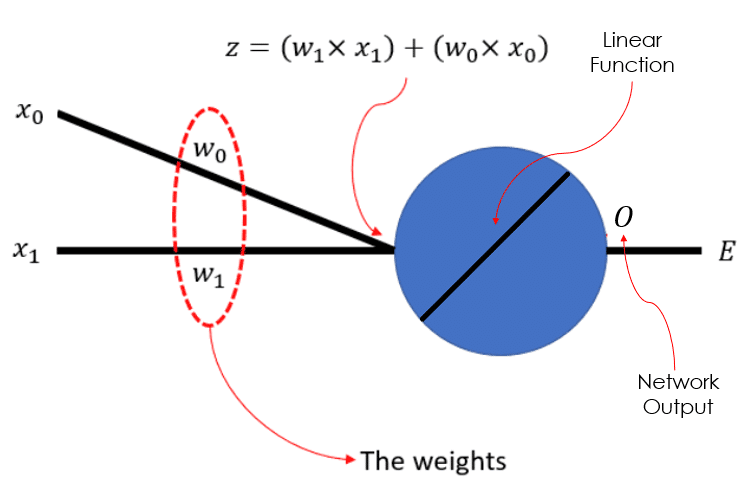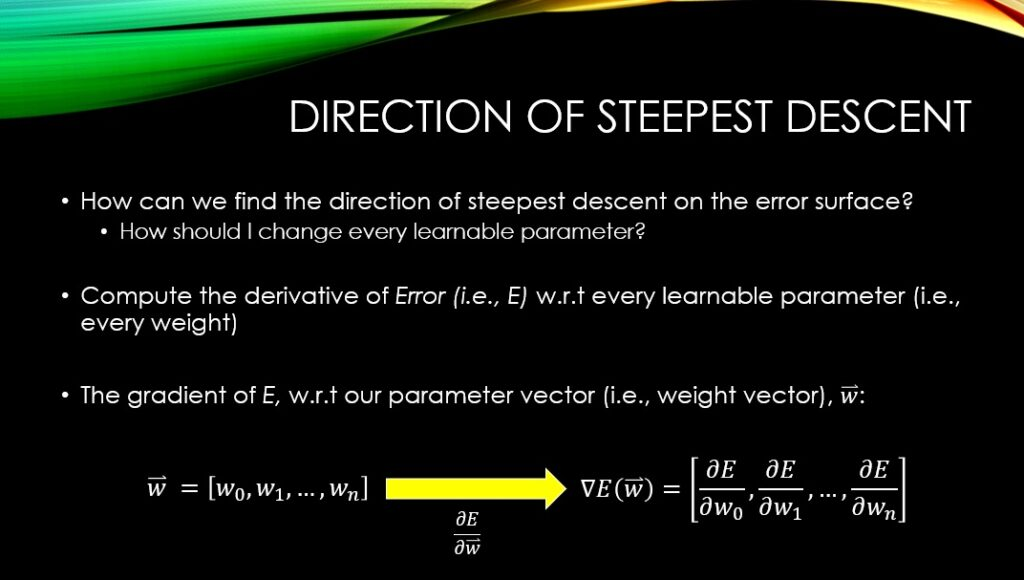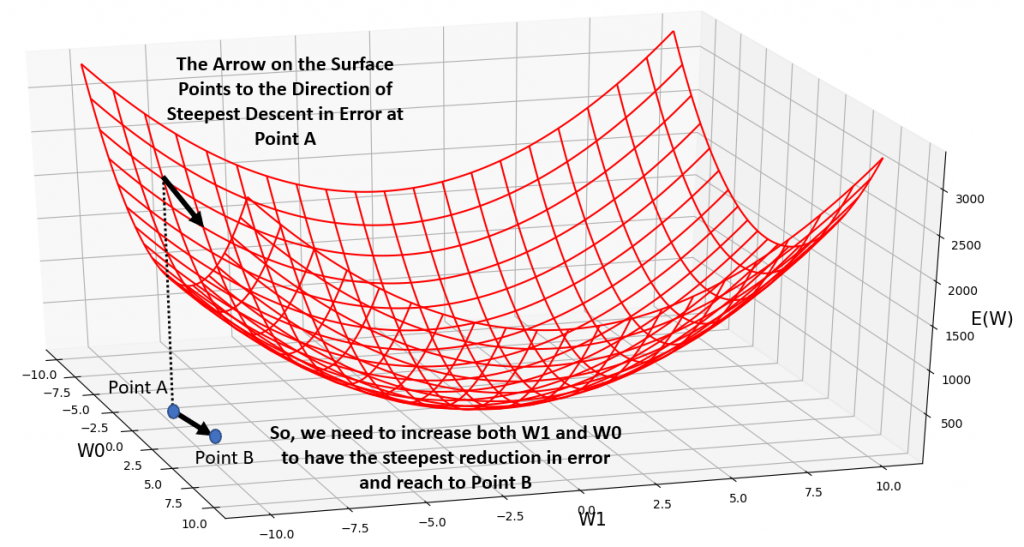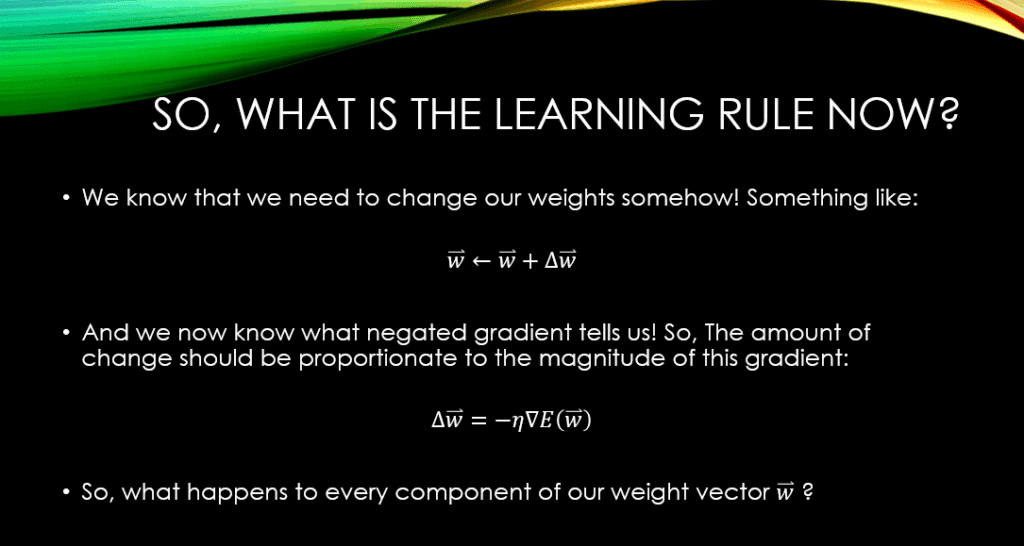# Deriving the Gradient Descent Rule (PART-1)

When training a model, we strive to minimize a certain error function (). This error function gives us an indication as to how well is our model doing on our training data. So, in general, the lower it is, the better our model is doing on the training set.

Make no mistake! We almost never want to keep training our model until the error is 0, or too small! Most likely, this would mean that the model has over-fitted (i.e., memorized) the training data. Thus, it will have a high generalization error when confronted with the unseen test data! So, we should be looking for a good local minima, and not a global minima!

So, for example in the case of training a neural network, we keep increasing and decreasing the weights inside our neural network, and search on the error surface. The question is:

How should we increase/decrease our weights (there could be millions of such weights in our neural network), to lower our training error (E) as much as possible!

So for example, consider the simple neural network down below, which has just 1 linear neuron, and 2 learnable weights:So, the question is how should we change the weights,and, so that our error,, would become suitably small. In other words:

Given a set of input data (say training data), some randomly chosen values for our weights, we can compute the resultant error using our error function (Means Square Error, Cross-Entropy, etc.). So how should we change our weights at every step to have the steepest descent in the value of our error (i.e., learning the patterns in the training data).

By taking the partial derivative of our error,, w.r.t each and every weight in our neural network, we can find the direction of the steepest ascent along the error surface.  So, if we havenumber of weights, by taking the derivative of, w.r.t each one of them, we will get the gradient vector which haselements as well.Let’s take a moment and understand what is happening here. Let’s consider the first element of the derivative vector,. It has a sign and it has a magnitude!

Let’s look at the magnitude first:

The magnitude ofshows the proportion of change inover, if we increase the current value ofa tiny bit! So, the higher this value, the more of a change inwill we observe, given a little increase in the current value of.

If, then it means that if we keep all the other weights constant, we will have to increaseto have moved along the direction of the steepest increase in, as far asis concerned! Similarly, if, then it means that if we keep all the other weights constant, we will have to decreaseto have moved along the direction of the steepest increase in, as far asis concerned.

So, if we know the direction and magnitude of change for every weight (i.e., increasing or decreasing them), using the gradient, we have moved towards the direction of the steepest ascent on the error surface, as far as (pay attention!!!) ALL OF OUR WEIGHTS, are concerned!

So what is the direction of steepest descent then? Of course, the negated direction of the gradient. So, the polar opposite of that!

## Visualizing the Direction of Steepest Descent

Take a look at the following error surface. The current point shows where we are on this surface, given the current values of our 2 weights (That we have shown in our simple neural network at the top of this page). The gradient w.r.t both of our weights is negative, so we need to increase both weights to move down-wards along the direction of the steepest descent on the error surface. So, you see that we are moving along the opposite direction of the gradient vector, by updating our weights.## Incorporating it All into the Gradient Descent Training Rule

It is now clear that, both the direction of change for every weight in our neural network, and the magnitude of that change, have some connection with the gradient of our error,, w.r.t to every one of those weights. As a result, for a given weight parameter,, we will compute an amount of change,(which is tightly tied with our gradient), and add it to our current value for.

We mentioned that we need to negatein order to move along the steepest descent on the error surface (rather than steepest ascent). So that is all about the direction of movement. Regarding the magnitude of movement, we know that the magnitude ofhas something to do with it!!! The higher it is, the more the error,, would change with a slight increase in the current value of our weight,. We tend to multiplyby a step size to determine the size of our step in changing our parameter,. This coefficient that we use for multiplication is called(pronounced ‘eta’), also known as the learning rate!

So we negate the value of the gradient, and then multiply the result by our learning rate, to see how much we should change our wights. This is all nicely summarized down below:## Conclusions

In this post we have learned about the importance of the gradient of our error function, with respect to our learnable parameters in a neural network. Especially, we have seen that both the sign and the magnitude of this gradient can help us determine how we should change (i.e., update) our weights, so that we would decrease the value of our error, the fastest. Hence, the name Gradient Descent!

In the next post, we will actually derive the gradient of an error function for our simple neural network, which has a linear neuron, with respect to our parameters. This will help you see what this gradient looks like, mathematically speaking!

Until then,

On behalf of MLDawn take care 😉